The new SAT requires you to know a number of special equation forms—to know which one you need to use in a given situation, and to know how to get into that form if it’s not the one you’re given by using algebraic manipulation. Some equation forms (vertex form of a parabola and the standard circle equation immediately spring to mind) contain binomial squares, e.g., as essential ingredients. To get a non-standard equation into these forms, you’ll often have to complete the square. I know, I know, you’ve done this a million times in school. Still, I often find students haven’t done this in a long time and need a little bit of a refresher. So here we are.

First, the equations in question.

Vertex form of a parabola: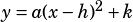, where the vertex of the parabola is at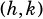.

Standard circle equation:, where a circle with radius r has its center at.

Say you’re given a parabola that’s not in vertex form and you need to put it in vertex form. How do you do that?

No calculator; grid-in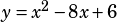The parabola formed when the equation above is graphed in the xy-plane has its vertex at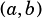. What is the value of?

Completing the square isn’t the only way to solve this question, but I’d argue it’s the fastest. All we need to do to go from the given form to the vertex form is figure out which binomial square thepart of the equation is the beginning of. With practice, this becomes second nature and you probably won’t need the rule, but the rule is that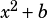is the beginning of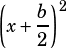.* In this case, that means thatis the beginning of.

Now, what do you get when you FOIL out? You get. That’s not what we have above—we haveinstead. Luckily, we can do anything we want to the right side of the equation provided that we keep the equation balanced by doing the same thing to the left, so we can just add 10 to both sides!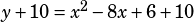From there, we’re almost done. Now we can convert the right side to the binomial square we wanted, and then get y by itself again to land in vertex form.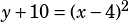So, there you have it: the parabola in question has a vertex of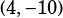. Since the question said the vertex was at, we know that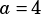,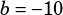, and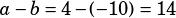. So, 14 is the answer.

Let’s practice with a few more, shall we? Try to do the following drill without a calculator. All three questions are grid-ins.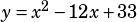The parabola formed when the equation above is graphed in the xy-plane has its vertex at. What is the value of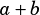?

Question 1 of 3

When the equationis graphed in the xy-plane, it forms a circle. What is x-coordinate of the center of the circle?

Question 2 of 3

What is the radius of the circle with equation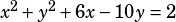?

Question 3 of 3

* I’m intentionally limiting this post to scenarios where the leading coefficient in the square being completed is 1. So far, I have not seen an official question of this type where that is not the case.Lucas Nguyen says:Mike McClenathan says:Lucas Nguyen says: